# Problem 57874. Easy Sequences 107: Minimized Circumcircle-Incircle Areas Ratio of Partial Pythagorean Triangles

We define a Partial Pythagorean Triangle (PPT) as a right triangle wherein the hypotenuse and at least one leg are integers. Thus, the triples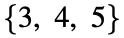and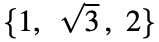represent a PPT, while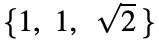,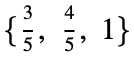and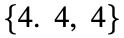do not.
Given the limit P, find the area of the PPT with perimeter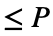, such that the ratio of the areas of the triangle's circumcircle to its incircle,, is as small as possible.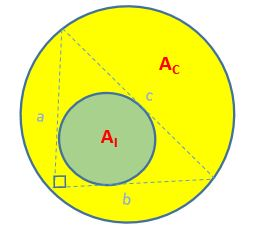### Solution Stats

0.0% Correct | 100.0% Incorrect
Last Solution submitted on Apr 26, 2023

#### Problem Recent Solvers0

No solvers yet, be the first player to solve this problem.

### Community Treasure Hunt

Find the treasures in MATLAB Central and discover how the community can help you!

Start Hunting!